# An Improved Model of Grinding

Our previous model assumed uniformly sized particles of ground coffee. However, real coffee grounds usually have a bimodal size distribution which looks something like this:

The size of the larger particles, often called “boulders”, is controlled by our grind setting. But there is also a peak on the left, corresponding with smaller particles called “fines”. The size of the fines doesn’t change much, but the number of fines increases as the grind size gets smaller.

Fines result from the fact that real coffee particles aren’t homogeneous. They are more like a dried out sponge, with internal pores surrounded by what used to be the cell walls of the coffee plant. Ground coffee particles are left with a lot of unsupported walls on their outer surface, which break off to form fines.

We can model this by dividing the spherical particle into a strong core and a brittle outer layer with a depth of about half the pore size.

From this model, we can get expressions for the mass of boulders and fines coming from a single particle of ground coffee:Here,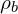is the density of the boulders;is the radius of the core; and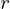is the thickness of the brittle layer.

We can also calculate the mass fraction of each particle species: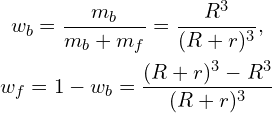At large grind sizes, as illustrated on the left below, the fines fit into the space between boulders. As grind size gets smaller, as shown on the right, those spaces become completely filled, and eventually the fines dominate the volume calculation, with boulders floating in a sea of fines.

When boulders dominate, the volume of our coffee grounds is just the volume of the packed boulders: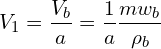Here,is the total volume of the boulders;is the packing density of the boulders; and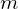is the total mass of ground coffee.

When fines dominate, the volume of our coffee grounds is the sum of the volume occupied by each type of particle: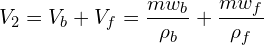Here,is the density of the packed fines. We can then calculate the density in each case:If we plot these two density curves, we get something like this:

The combined density curve is shown as a dashed black line. We can fit this curve to our data by lettingbe equal to our grind setting, then adjusting,,, andso that the error between the curve and our density measurements is minimized. Here’s what that looks like:

It’s a little surprising how good this fit is—it even accounts for the small “bump” in the data. What’s really interesting is that this fit allows us to infer some microscopic properties of the ground coffee.

The packing density of the boulders () is 0.81. This is a little higher than I would expect, since randomly packed spheres usually have a packing density around 0.64. However, it could be that, e.g., the irregular surface of the boulders is affecting this value.

The density of the boulders () is 0.50 g cm-3. This is typical for roasted coffee beans. Note that the density of the boulders is the same as the density of the roasted beans.

The density of the fines () is 0.66 g cm-3. This is denser than the boulders, which we might expect given the irregular shape of the fines.

The thickness of the brittle layer () is 0.17 grind steps. On the Eureka Mignon Specialita, used here, each grind step is about 50 μm, which suggests the brittle layer is about 8.5 μm thick on average. It’s tempting to infer that the pore size is about 17 μm, but I think this would be incorrect.

How much of the brittle layer breaks off will depend on the grinding process. If the brittle layer breaks off over half the surface area of the ground particles, then the calculated average thickness will be half of what we might expect it to be. Because of this, I think this figure may be more useful in comparing grinders, when the same beans are used in both grinders.

Knowing the average thickness of the brittle layer, we can compute the mass fraction of fines and boulders as a function of grind setting, using the equations above. This results in the following plot, for the beans and grinder used in this experiment:

Finally, it’s worth noting that the properties we’ve inferred here seem reasonable, but in order to validate the model we should measure these properties in some other way, and then compare those results against the results we’ve obtained here.

Correction: In the original post, I defined the density of the boulders and fines as: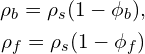Here,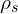is the density of the solid coffee—i.e., the solid part of the sponge;is the porosity of the coffee bean—i.e., how much of its volume is occupied by pores; and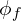is the porosity of the packed fines.

However,,, andonly occur in these combinations, so we cannot separate them without more information. Therefore, I’ve updated the post to useandinstead.